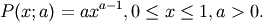# numpy.random.mtrand.RandomState.power¶

RandomState.power(a, size=None)

Draws samples in [0, 1] from a power distribution with positive exponent a - 1.

Also known as the power function distribution.

Parameters: a : float parameter, > 0 size : tuple of ints Output shape. If the given shape is, e.g., (m, n, k), then m * n * k samples are drawn. samples : {ndarray, scalar} The returned samples lie in [0, 1]. ValueError : If a<1.

Notes

The probability density function isThe power function distribution is just the inverse of the Pareto distribution. It may also be seen as a special case of the Beta distribution.

It is used, for example, in modeling the over-reporting of insurance claims.

References

 [R218] Christian Kleiber, Samuel Kotz, “Statistical size distributions in economics and actuarial sciences”, Wiley, 2003.
 [R219] Heckert, N. A. and Filliben, James J. (2003). NIST Handbook 148: Dataplot Reference Manual, Volume 2: Let Subcommands and Library Functions”, National Institute of Standards and Technology Handbook Series, June 2003. http://www.itl.nist.gov/div898/software/dataplot/refman2/auxillar/powpdf.pdf

Examples

#### Previous topic

numpy.random.mtrand.RandomState.poisson

#### Next topic

numpy.random.mtrand.RandomState.rand